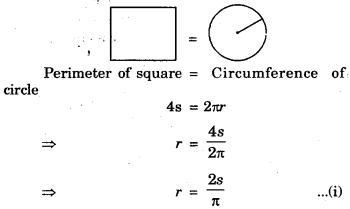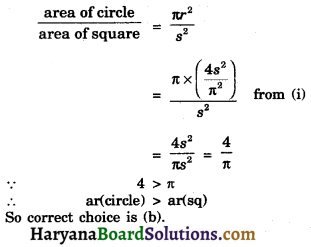# HBSE 10th Class Maths Important Questions Chapter 12 Areas related to Circles

Haryana State Board HBSE 10th Class Maths Important Questions Chapter 12 Areas related to Circles Important Questions and Answers.

## Haryana Board 10th Class Maths Important Questions Chapter 12 Areas related to Circles

Question 1.
Find the area of a quadrant of a circle whose circumference is 44 cm.
Solution :
Let the radius of a circle be r cm.
The circumference of a circle = 44 cm (given)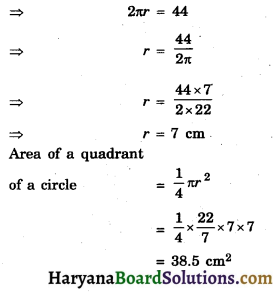Question 2.
The short hand and long hand of clock are 4 cm and 6 cm long respectively. Find the sum of distances travelled by their tips in 48 hours.
Solution :
The distance travelled by shorthand in 12 hours = 2πr
= 2 × π × 4
[∴ r = 4 cm]
The distance travelled by shorthand in 48 hours = 4 × 8x
= 32πcm
The distance travelled by long hand in 12 hours = 4 × 2лR
= 4 × 2π × 6
[∴ R = 6 cm]
Total distance travelled by their tips in 48 hours = 32π + 48л
= 80π cm.Question 3.
If the angle of a major sector of a circle is 250°. Then find the angle of minor sector.
Solution :
We have
Angle of major sector = 250°
∴ Angle of minor sector = 360° – 250° = 110°

Question 4.
If an arc of a circle subtends an angle of 60° at the centre and if the area of minor sector is 231 cm2, then find the radius of the circle.
Solution :
We have sector angle (θ) = 60°
Let radius of circle be r cm
Area of minor sector = 231 cm2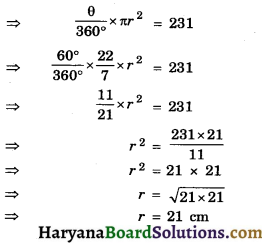Hence, radius of the circle = 21 cmQuestion 5.
The perimeter of a sector of a circle of radius 5.2 cm is 16.4 cm. Find the area of the sector.
Solution :
We have
Radius of sector of a circle (r) = 5.2 em
Let sector angle be θ
Perimeter of a sector of a circle = 16.4 cm (given)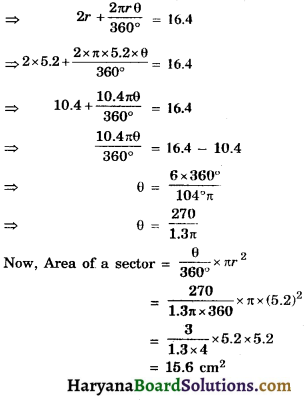Question 6.
Find the area of sector of a circle of radius 6 cm whose central angle is 30°. (take π = 3.14)
Solution :
We have
Radius of a circle (r) = 6 cm
Sector angle (θ) = 30°
Area of a sector = $$\frac{\pi^2 \theta}{360^{\circ}}$$
= $$\frac{3.14 \times 6^2 \times 30^{\circ}}{360^{\circ}}$$
= 3.14 × 3
= 9.42 cm2

Question 7.
In the given figure is a sector of circle of radius 10.5 cm. Find the perimeter of sector.
(Take π = 22/7)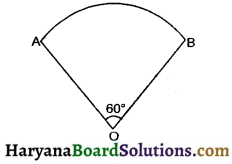Solution :
We have
Radius of a sector of circle (r) = 10.5 cm
Sector angle (θ) = 60°
Perimeter of sector = $$\frac{2 \pi r \theta}{360^{\circ}}$$
= $$\frac{2 \times 22}{7} \times \frac{10.5 \times 60}{360^{\circ}}$$
= 22 × 0.5
= 11 cm2Question 8.
In the given figure, AOB is a sector of angle 60° of a circle with centre O and radius 17 cm. If AP ⊥ OB and AP = 15 cm, find the area of shaded region.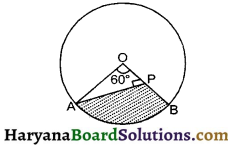Solution :
We have
Radius of circle (r) = AO 17 cm
∠APO = 90° and AP = 15 cm,
sector angle (θ) = 60°
In right triangle AOP
⇒ AO2 = AP2 + OP2
172 = 152 + OP2
172 – 152 = OP2
(17 + 15) (17 – 15) = OP2
32 × 2 = OP2
OP = $$\sqrt{m}$$ = 8 cm
= Area of sector AOBA – area of Δ AOP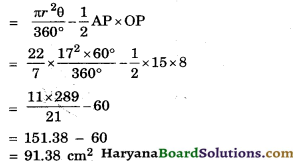Question 9.
In the given figure two arcs, A and B. Are A is part of the circle with centre O and radius OP. Arc B is part of the circle with centre M and radius PM, where M is the midpoint of PQ. Show that area enclosed by two arcs is equal to ($$\sqrt{3}$$ – $$\frac {π}{6}$$) cm2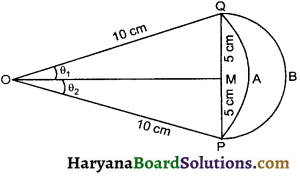Solution :
We have
R = 5 cm
Area of semicircle (A1) = $$\frac {1}{2}$$πR²
= $$\frac {1}{2}$$ × π × 52
= $$\frac {25π}{2}$$ cm2
Let ∠MOQ = ∠MOP = Q1
In the right ΔOMQ, we have
sin θ1 = $$\frac{\mathrm{MQ}}{\mathrm{OQ}}=\frac{5}{10}=\frac{1}{2}$$
sin θ1 = sin 30°
θ1 = 30°
∠POQ = 2θ = 2 × 30° = 60°
Area enclosed by are A and chord PQ (A2)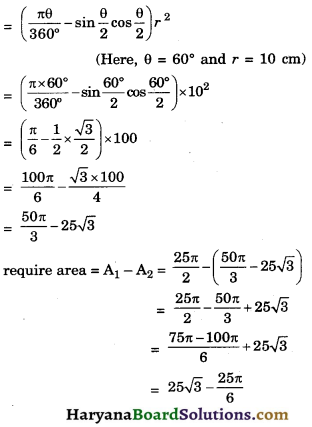= 25($$\sqrt{3}$$ – $$\frac {π}{6}$$)
So, required area = 25($$\sqrt{3}$$ – $$\frac {π}{6}$$)
Hence ProvedQuestion 10.
Find the area of shaded region in the givem figure, where arcs drawn with centres A, B, C, D intersect in pairs at midpoints P, Q, Rand S of the sides AB, BC, CD and DA respectively of square ABCD of side 12 cm.
[use π = 3.14]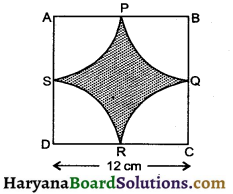Solution :
We have
side of a square = 12 cm
Area of a square (ABCD)= 12 × 12 = 144 cm2
Radius of each sector (r) = $$\frac {12}{2}$$ = 6cm
Each sector angle (θ) = 90°
Area of each sector = $$\frac{\pi r^2 \theta}{360^{\circ}}$$
= $$\frac{3.14 \times 6^2 \times 90}{360^{\circ}}$$
= 3.14 × 9
= 28.26 cm2
Area of 4 sectors = 28.26 × 4
= 113.04 cm2
= Area of square – 4 sectors area
= 144 – 113.04
= 30.96 cm2
= 7 cm

Question 11.
In the adjoining figure ABCD is a trapezium with AB || DC and ∠BCD = 30°. In figure BGEC is a sector of a circle with centre C and AB = BC = 7 cm, DE = 4 cm and BF = 3.5 cm, find the area of the shaded region. [use x = $$\frac {22}{7}$$]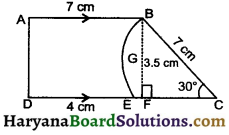Solution :
We have
AB = 7 cm, DE = 4 cm and BF = 3.5 cm
DC= DE + EC = 4 + 7 = 11 cm
Area of trapezium ABCD
= $$\frac {1}{2}$$(AB + DC) × BF
= $$\frac {1}{2}$$(7 + 11) × 3.5
= 9 × 3.5
= 31.5 cm2
Area of sector BGEC = $$\frac {θ}{360°}$$ πr²
= $$\frac {30°}{360°}$$ × $$\frac {22}{7}$$ × 72
= $$\frac{22 \times 7}{12}$$
= 12.83 cm2
Area of shaded region = Area of trapezium – sector area
= 31.5 – 12.83
= 18.67 cm2Question 12.
The side of a square is 10 cm, find the area between inscribed and circumscribed circle of the square.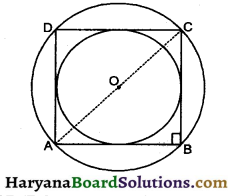Solution:
Let ABCD be a square of side 10 cm
Radius of inscribed circle (r) = 5 cm
∴ Area of inscribed circle (A1) = πr²
= π × 52
= 25π cm2
In right ΔABC, we have
AC2 = AB2 + BC2
AC2 = 102 + 102
AC2 = 100 + 100
AC = 200
AC = $$\sqrt{2 \times 10 \times 10}$$ = 10$$\sqrt{2}$$ cm
Radius of circumscribed circle (R) = $$\frac{10 \sqrt{2}}{2}$$
= 5$$\sqrt{2}$$
Area of a circumscribed circle (A2) = πR²
= π × (5$$\sqrt{2}$$)2
= 50π
Required area = A2 – A1
= 50π – 25π
= 25π cm2

Question 13.
In the give figure, the boundary of the shaded region consists of four semicircular ares, the smallest two being equal. If the diameter of the largest is 14 cm and of the smallest is 3.5 cm, find.
(i) length of the boundary
(ii) the area of the shaded region.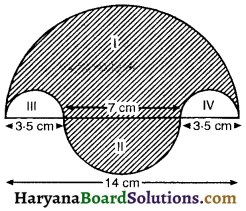Solution :
We have,
Diameter of the biggest circle = 14 cm
∴ radius of the biggest circle (r1) = $$\frac {14}{2}$$ = 7 cm and diameter of the smallest circle = 3.5 cm
∴ radius of the two smallest circle (r2) = $$\frac {3.5}{2}$$
and radius of the circle (II) = r3 = $$\frac {7}{2}$$ = 3.5 cm
(i) length of the boundary = πr1 + 2 × πr2 × πr3
= $$\frac {22}{7}$$ × 7 + 2 × $$\frac {22}{7}$$ × $$\frac {3.5}{2}$$ + $$\frac {22}{7}$$ × 3.5
= $$\frac {22}{7}$$ [7 + 3.5 + 3.5]
= $$\frac {22}{7}$$ × 14
= 44 cm

(ii) The area of the shaded region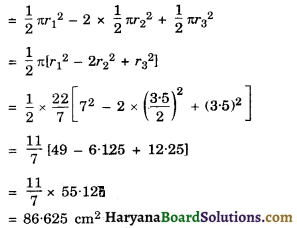Hence, (i) length of the boundary = 44 cm
(ii) Area of the shaded region = 86.625 cm2Question 14.
In the given, the side of square is 28 cm, and radius of each circle is half of the length of the side of the square, where O and O’ are centers of the circle. Find the area of shaded area.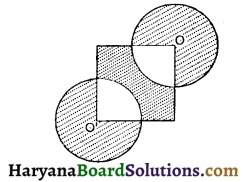Solution :
Side of the square = 28 cm (given)
∴ Area of sqaure = 28 × 28 = 784 cm2
∴ Radius of each circle is half of the length of the side of the square (given)
∴ Radius of each circle (r) = $$\frac {1}{2}$$ × 28 = 14cm
Area of two circles = 2πr²
= 2 × $$\frac {22}{7}$$ × 142
= 44 × 2 × 14
= 1232 cm2
Area of two quadrants = 2 × $$\frac{\pi r^2 \theta}{360^{\circ}}$$
= 2 × $$\frac {22}{7}$$ × $$\frac{14^2 \times 90}{360}$$
= 44 × 7
= 308 cm2
Area of shaded region = Area of square + area of two circles – area of two quadrants
= 784 + 1232 – 308
= 1708 cm2

Question 15.
In the given figure, Δ ABC is right angles triangle in which ∠A = 90°. Semicircles are drawn on AB, AC and BC as diameters. Find the area of shaded region.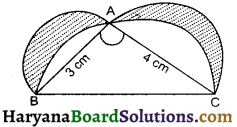Solution :
In Δ ABC, we have
∠A = 90°, AB = 3 cm, AC = 4 cm
BC2 = AB2 + AC2
[By Pythagoras theorem]
⇒ BC2 = 32 + 42
= 9 + 16 = 25
BC = 5 cm
Area of semicircle with radius $$\frac {3}{2}$$ cm (A1)
= $$\frac {1}{2}$$π × ($$\frac {3}{2}$$)2 = $$\frac {9}{8}$$π cm2
Area of semicircle with radius $$\frac {4}{2}$$ cm (A2)
= $$\frac {1}{2}$$π × 22 = $$\frac {4π}{2}$$་cm2
Area of semicircle with radius $$\frac {5}{2}$$ cm (A3)
= $$\frac {1}{2}$$π × ($$\frac {5}{2}$$)2
= $$\frac {25}{8}$$π cm2
Area of right triangle ABC = $$\frac {1}{2}$$ × 3 × 4 = 6cm2
area of shaded region = A1 + A2 – (A3 – A4)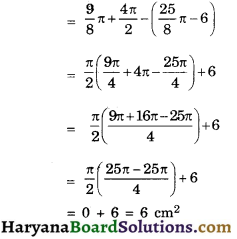Question 16.
Sides of a triangular field are 15 m, 16 m and 17. With the three corners of the field a cow, a uffalo and a horse are tied separately with ropes of length 7 m each to graze in the field. Find the area of the field which cannot be grazed by the three animals.
Solution :
We have,
Radius of each sector is = 7 m
Sides of triangle are 15 m, 16 m and 17 m respectively.
Let a cow, a buffalo and a horse are tied respectively with vertex A, B and C separately.
Let ∠A = x1°, ∠B = x2°, ∠C = x3°
The area of the field which can graze by three animals Sum of the areas of three sectors with sector angles x1°, x2°, x3° and radius 7m.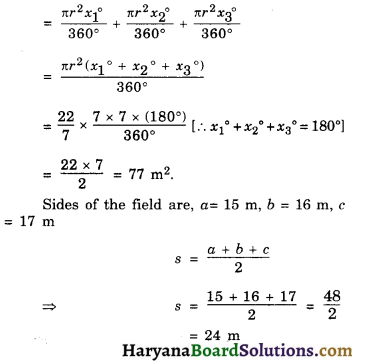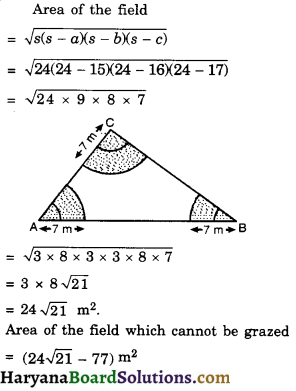Question 17.
In the adjoining figure, O is the centre and BOC is the diameter of the circle. Find the area of the shaded region. (use π = 3.14)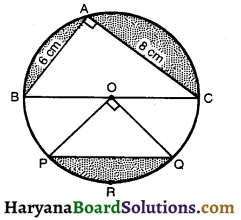Solution :
We have,
AB = 6 cm, AC = 8 cm and BOC is the diameter of the circle.
In right ΔBAC,
BC2 = AB2 + AC2
[By Pythagoras theorem]
BC2 = 62 + 82
BC2 = 36 + 64
BC2 = 100
BC = $$\sqrt{100}$$ = 10 cm.
Radius of the circle = $$\frac {10}{2}$$ = 5 cm.
Therefore,
OP = OQ = 5 cm
Shaded region area = Semi-circle area drawn as BC diameter – ΔABC area + segment PRQ area
= $$\frac {1}{2}$$π × (5)2 – $$\frac {1}{2}$$ × 6 × 8 + area of quadrant POQ – area of ΔPOQ
= $$\frac {1}{2}$$ × 3.14 × 25 – 24 + $$\frac {1}{4}$$π × (5)2 – $$\frac {1}{2}$$ × 5 × 5
= 39.25 – 24 + $$\frac {1}{4}$$ × 3.14 × 25 – 12.5
= 15.25+ 19.625 – 12.5
= 34.875 – 12.5
= 22.375 = 22.38 cm2. (approx)
Hence, area of shaded region = 22.38 cm2.
(approx)

Fill in the Blanks

Question 1.
The region between an are and the ……….. joining the centre to the end points of the arc is called a sector of the circle.
Solution :Question 2.
A ……….. of a circle is a line segment joining any two points on the circle.
Solution :
chord

Question 3.
The length of the complete circle is called its……….
Solution :
circum- ference

Question 4.
The region between a chord and either of its …………. is called a segment of the circle.
Solution :
src

Question 5.
The cicles which have same centre and different …………. are called concentric circle.
Solution :Question 6.
If the perimeter of a circle is equal to that of a square, then the ratio of their areas is ……..
Solution :
14 : 11

Multiple Choice Questions

Choose the correct answer for each of the following :

Question 1.
The circumferences of two circles are in the ratio 3 : 4. The ratio between their areas is:
(a) 9 : 4
(b) 16 : 9
(c) 3 : 4
(d) 9 : 16
Solution :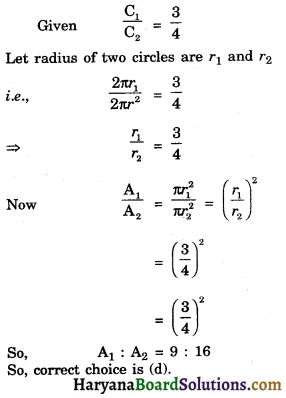Question 2.
The areas of two circles are in the ratio 16 : 25. The ratio of their circumference is:
(a) 4 : 5
(b) 5 : 4
(c) 16 : 25
(d) 25 : 16
Solution :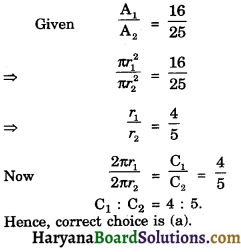Question 3.
The area of a circle is 13.86 cm2, the circumference of the circle is :
(a) 13.6 cm
(b) 13.2 cm
(c) 6.6 cm
(d) 13.4 cm
Solution :
(b) 13.2 cm

Given, area of circle 13.86 cm2
⇒ πr² = 13.86
3.14 r² = 13.86
r² = $$\frac {13.86}{3.14}$$
r = 2.1 cm
∴ circumference = 2πr
= 2 × $$\frac {22}{7}$$ × 2.1
= 13.2 cm
So correct choice is (b).Question 4.
Area of the largest triangle that can be inscribed in a semicircle of radius r units is :
(a) r2 sq. units
(b) $$\frac {1}{2}$$r2 sq. units
(c) 2r2 sq. units
(d) $$\sqrt{2}$$r2 sq. units
Solution :
(a) r2 sq. units

Area of triangle = $$\frac {1}{2}$$base × height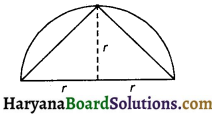= $$\frac {1}{2}$$ × 2r × r
= r2 sq. units.
Hence correct choice is (a).

Question 5.
The area of sector of central angle of a circle with radius 4r is: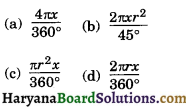Solution :
Area of sector = $$\frac{\pi r^2 \theta}{360^{\circ}}$$
= $$\frac{\pi(4 r)^2 x}{360}$$
= $$\frac{2 \pi \cdot x r^2}{45^{\circ}}$$
Hence, correct choice is (b).Question 6.
If the sum of the circumference of two circles with radii R1 and R2 is equal to the circumference of a circle of radius R, then :
(a) R1 + R2 = R
(b) R1 + R2 > R
(c) R1 + R2 < R
(d) Nothing definite can be said about the relation among R1, R1 and R.
Solution :
(a) R1 + R2 = R

2πR = 2πR1 + 2πR2
2πR = 2 × (R1 + R2)
R = R1 + R2
Hence correct choice is (a).

Question 7.
If the perimeter of circle is equal to that of a square, then ratio of their area is
(a) 22 : 7
(b) 14 : 11
(c) 7 : 22
(d) 11 : 14
Solution :
(b) 14 : 11

Perimeter of circle = 2πr
Perimeter of square = 4a
A.T.Q. 2π = 4a
side of square a = $$\frac {πr}{2}$$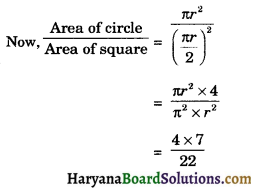So required ratio = 14: 11
So correct choice is (b).Question 8.
If the circumference of a circle and the perimeter of a square are equal, then :
(a) Area of circle = Area of the square
(b) Area of circle > Area of the square
(c) Area of circle < Area of the square
(d) Nothing definite can be said about the relation between the areas of the circle and square
Solution :